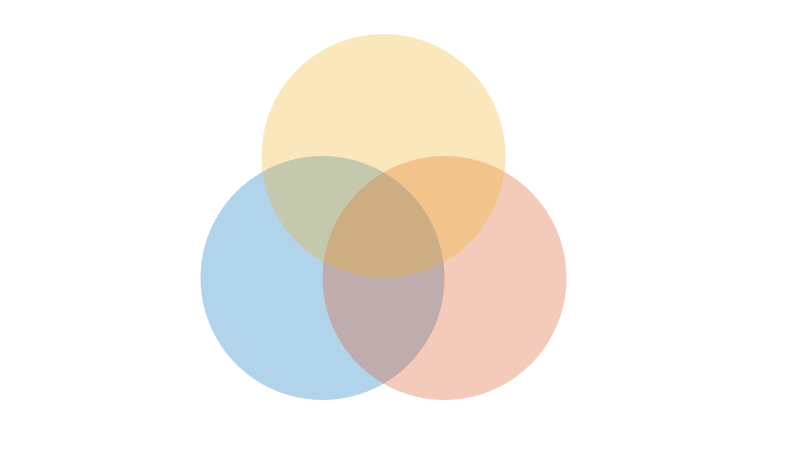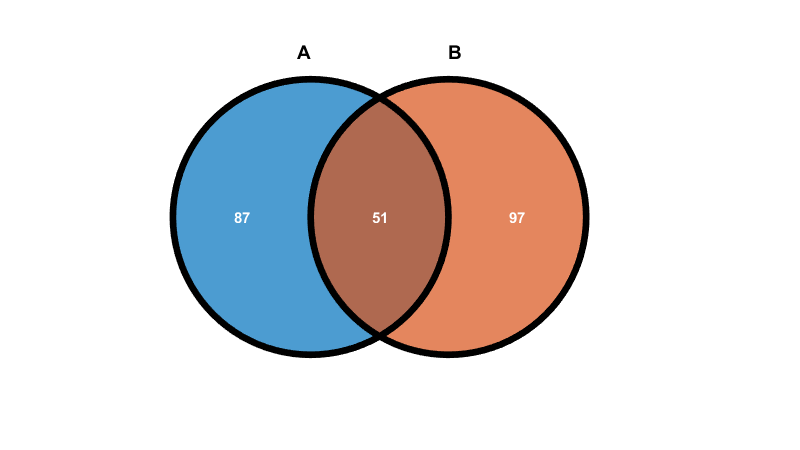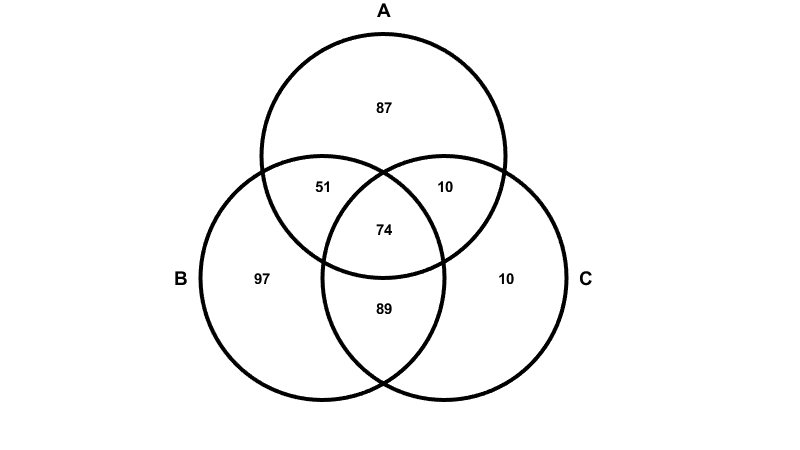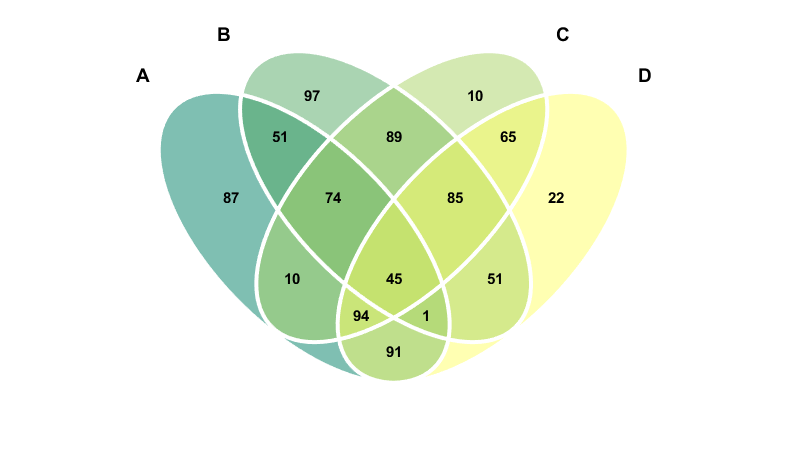## Venn diagram

バージョン 1.0.0 (143 KB) 作成者:
venn: A simple MATLAB function to draw Venn diagram of two to four sets with optional labels.

ダウンロード: 44

GitHub から

# venn: A simple MATLAB function to draw Venn diagram of two to four sets with optional labels

User can specify the number of sets to draw (maximum four) and label each set and the intersectional regions between sets.

Man Ho Wong, 2022.

## Installation

Requirement: MATLAB R2007a or above.

User can download the file `venn.m` in this repository directly or intall the function via MATLAB's Add-On Explorer.

## Usage

``````Input : n [positive integer]
Number of sets to draw
sets [string | char | cellstr | numeric]
An array of set names in left-to-right order
labels [string | char | cellstr | numeric]
An array of label names for labeling each section;
For Set A, B, C and D, there are 15 sections: A, B, C, D,
A&B, A&C, A&D, B&C, B&D, C&D, A&B&C, A&B&D, A&C&D, B&C&D,
A&B&C&D. Elements in 'labels' must follow the above order,
e.g. for Set A and B, the label order should be A, B,
A&B; Any extra labels will be ignored.
colors [rows of RGB triplet]
Color map for fill colors in left-to-right order.
e.g. [1 0 0; 0 1 0; 0 0 1] represents red, green, blue;
If number of colors is less than n, colors will be
repeated.
alpha [0 to 1]
Fill color transparency; 0 = fully transparent.
edgeC [RGB triplet]
Edge color (only effective when 'edgeW' is > 0).
edgeW [positive number]
Edge width (By default, there is no edge)
labelC [RGB triplet]
Color of section labels.

Output : A Veenn diagram will be drawn on a new figure.
vennfig (optional): A handle to the figure.
``````

## Examples

Draw three sets with default settings:

``````venn(3);
``````Assign names and use random integers as section labels:

``````mysets = ["A" "B" "C" "D"];
mylabels = randi(100,[15,1]);
``````

Draw two sets with labels; set fill alpha (transparency) to 0.7, set label color to white and use black edges with custom thickness:

``````venn(2,sets=mysets,labels=mylabels,alpha=0.7,edgeC=[0 0 0],labelC=[1 1 1],edgeW=5);
``````Draw three sets with labels; set fill alpha to 0 and use black edges with custom thickness:

``````venn(3,sets=mysets,labels=mylabels,alpha=0,edgeC=[0 0 0],edgeW=3);
``````Draw four sets with labels; use custom color map, set alpha to 0.5 and use white edges with custom thickness:

``````c = summer(4);
venn(4,sets=mysets,labels=mylabels,colors=c,alpha=0.5,edgeC=[1 1 1],edgeW=3);
``````## Cite As

Wong, M. H. (2022). venn: A simple MATLAB function to draw Venn diagram of two to four sets with optional labels. (1.0.0). Zenodo. https://doi.org/10.5281/zenodo.7297812

### 引用

Man Ho Wong (2023). Venn diagram (https://github.com/manhowong/matlab-venn/releases/tag/v1.0.0), GitHub. 取得済み .

Wong, Man Ho. Venn: A Simple MATLAB Function to Draw Venn Diagram of Two to Four Sets with Optional Labels. Zenodo, 2022, doi:10.5281/ZENODO.7297812.

その他のスタイルを見る
##### MATLAB リリースの互換性

R2007a 以降のリリースと互換性あり
##### プラットフォームの互換性
Windows macOS Linux

### Community Treasure Hunt

Find the treasures in MATLAB Central and discover how the community can help you!

Start Hunting!
バージョン 公開済み リリース ノート
1.0.0

この GitHub アドオンでの問題を表示または報告するには、GitHub リポジトリにアクセスしてください。
この GitHub アドオンでの問題を表示または報告するには、GitHub リポジトリにアクセスしてください。